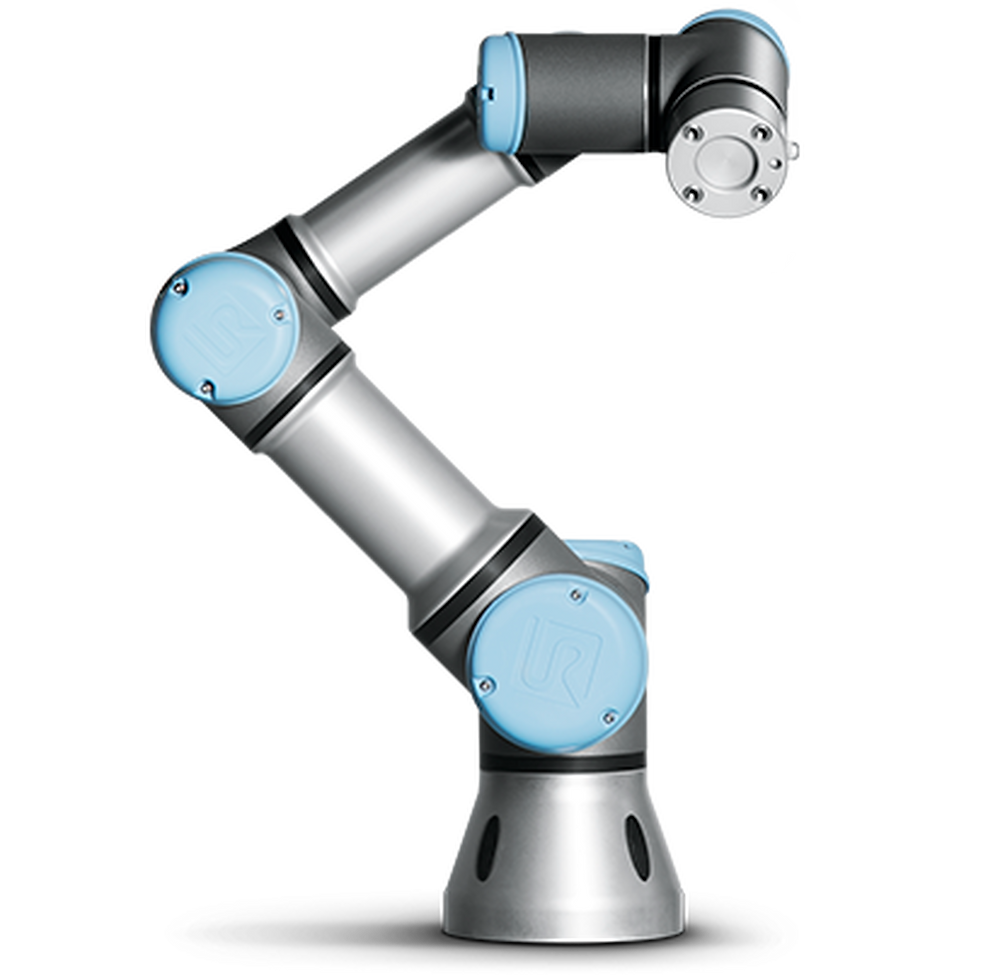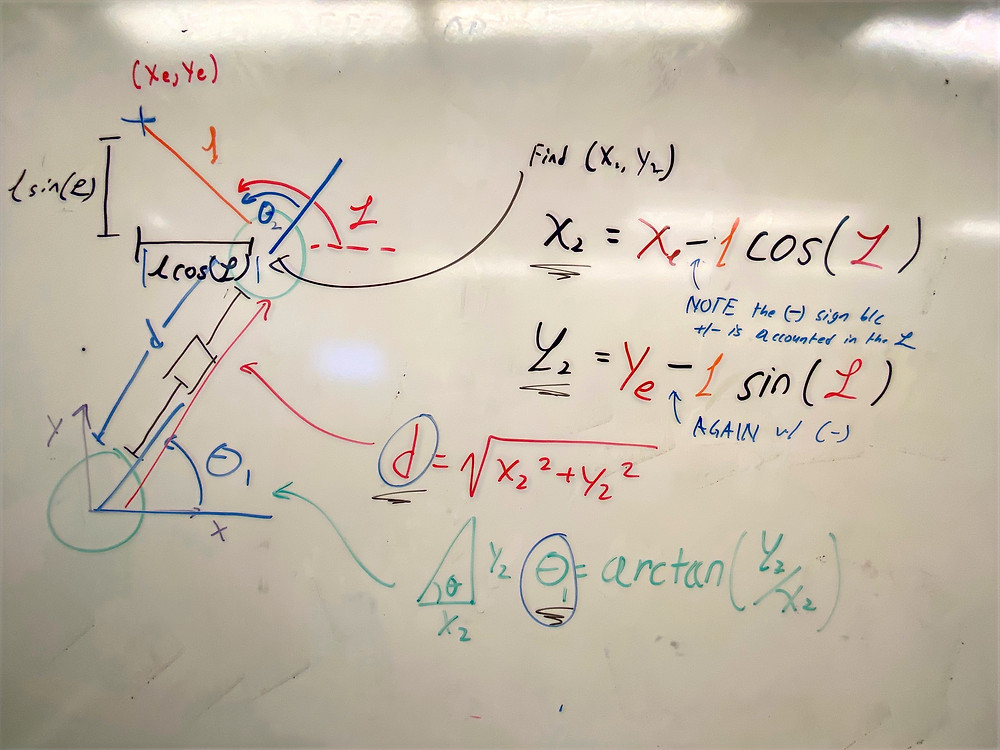Search
• aditya mehrotra.

# inverse kinematics for dummies by dummies [robotics must-knows]

Updated: Feb 11, 2020

So todayyy we're going to do two long-ish posts. This one, about what actually is inverse kinematics now that I've learned a good amount about it in 2.12. This post is basically my 2.12 lecture from today! And the post after this will be the plan for how we are actually modeling and controlling the scout leg system using inverse kinematics, the plan for zeroing and etc. We tried doing some IK posts earlier but it didn't really work because I was too dumb to know where to look to learn it. But it was explained to me really well today so we shall start from the beginning!

## some basic vocabulary and background:

So a robot, essentially, is made of many different components at the system-level. But at its core, you have a bunch of joints, and a bunch of things that connect these joints. Let's look at this robot arm below.As we can see in the above diagram, this robot arm has joints (which are the motors), and straight sections that connect them (the arms). At the end is what's called an End Effector (that is just the tool that the robot uses at the end of the arm). We generally care mostly, about the position of this End Effector.

There are two types of robotics joints/motion components. We can have revolute joints which are like motors and traditional spinning actuators. They ROTATE. Then we have prismatic joints which are like linear actuators and straight line motion components, as diagramed below.So these are the basic terms you'll need to know to understand the rest of this post. There's the revolute joint, the prismatic joint, and the end-effector. Now it's time to start examining Kinematics & Inverse Kinematics (K & IK).

## what is kinematics:

So kinematics, for those of you who know some basic physics, is the idea that we take a system and based on giving things a certain velocity or moving them to a certain position we can describe the location of points in space. In English - if I make something move, how do I calculate where it goes when I've moved it. That's kinematics. And it's best explained with an example. Let's take a look at the robot arm we were looking at before but this time let's add one more prismatic joint.So we have a revolute joint at the bottom, a prismatic joint as the connection between the first and second revolute joint, a revolute joint in the middle, and a rigid length arm connecting the second revolute joint to the end effector. So the first thing we need to do is define a few variables that will allow us to describe this system. Let's start by defining the global coordinate system. We're just going to set this to the "up" and the "right" direction where the origin the the first joint/actuator. We're also going to define three quantities to define the state of the three joints in the system. THETA_1, d, and THETA_2 which are the rotational position of the first actuator with respect to the x-axis, the length of the second actuator, and the rotational position of the third actuator with respect to the line between the first and second actuator. Does that make sense? It's important to understand what the inputs to the system are and these are them. These three values describe what we would send the robot's motors to achieve this position, makes sense?Now if we have THETA_1, d, and THETA_2 we can find the position of the End Effector, which is what we care about as a part of this controls problem right? We want to know where that is at all times because that's what we are trying to control. Let's define some quantities to describe that, x_e, y_e, and an angle from the x-axis PHI. These quantities represent the x-position, y-position, and orientation from the x-axis with respect to the GLOBAL ORIGIN.So THETA_1, d, and THETA_2 are considered the inputs of the system, right? These are what we actually send to the robot arm. If the robot arm is considered the system, the outputs of the system, then, are x_e, y_e, and PHI, right? Because that's what we care about. If we're trying to pick up an object we care about where the gripper is in this 2-D space case!

Now what we can do is write some equations to go from THETA_1, d, and THETA_2 to x_e, y_e, and PHI. This is what we mean when we say forward kinematics.

Now let's actually do some math. This should be a somewhat simple geometry problem given the variables we've been given. We won't go through the full derivation in this post, we'll give you the answers and hopefully from the diagrams above you'll be able to derive the forward kinematic equations. We will go through the full derivation of inverse-kinematics, however.If you're confused at how we got these equations, you may want to look at this video. It really is trigonometry to get the FK equations.

## now, inverse kinematics, yay:

Okay so the question is, what in the world is inverse kinematics. WHY would I ever want to inverse a perfectly good kinematic. What's the points right? I know where my robot is going to go if I give it certain control inputs. Isn't that all I need. NO. What's more useful is NOT the answer to the question: "where will the end effector go if I move the joints in this way?" it's the question of: "how do I need to move the joints to get my end effector to go here?" SEE the difference? One asks how do I get x_e, y_e, and PHI from THETA_1, d, and THETA_2. The true question we want to answer is if I know what I want x_e, y_e, and PHI to be (for example, if I want to move the gripper to a certain position and orientation to pick up a ball in a known location), how do I set THETA_1, d, and THETA_2 to do that (how do I drive the motors to do that)? Now that's more interesting...

Let's take a look at that diagram again, with the outputs and inputs of the system labeled.What we are going to attempt to do, is find equations for THETA_1, d, and THETA_2 based on a known x_e, y_e, and PHI. One way to do this is develop the FK equations for the system like we did above, and plug in the desired x_e, y_e, and PHI and solve the system of equations. This is usually how we will do it in a robotics algorithm. Let's do it analytically for the sake of learning.

The first thing to realize is we know the position of the first actuator is (0,0) and we can obtain the position of the second actuator. Note how we did this - again it's simple geometry!Now that we have the position of the second actuator with respect to the origin (which is the first actuator). Right? And since we have the position of that - look! We can now get the distance "d" needed and the angle "THETA_1" needed!And now... since we have THETA_1, we can also get THETA_2 and the system is solved!So that's how we get the required motions of the actuators given a desired end-effector position, or how to do inverse kinematics. If you're still confused, this video is worth watching.

## further reading and etc:

So the above example is a very basic inverse-kinematics example. The idea here was to introduce the concept but when we start getting into 3D space and etc, inverse kinematics gets really difficult. That's reading for another time. Or for your googling purposes. For now this should get you to understand what inverse kinematics is and why it's important for robots.

Before we end I wanna clarify one thing. Let’s say you have a legged robot - Inverse Kinematics does NOT tell you how to move the legs and in what trajectory to move them to make the dog walk or something. That’s a separate modeling problem likely solved by motion profiling. No - IK is what you use AFTER you’ve gotten the trajectory and you use IK to get the actuation commands to follow that trajectory. Let’s say the trajectory contains 6 points, you do IK on each of the 6 points to know how to move the actuators. But ALSO note that IK does NOT move the actuators themselves - it tells you HOW to move them but not with what speed or etc. Usually you send the outputs of IK to a PID controller or feedback control system that actually moves the actuators to their places.

So in summary - inverse kinematics is the math between knowing where you want the robot to go, and actually moving the robot to that location.

4 views0 comments

### Recent Posts

See All

#### chip updates: combining a kangaroo, a ship pulley, and a wild robot arm into a leg [updates]

© copyright 2019 | aditya mehrotra